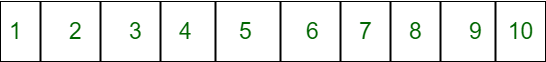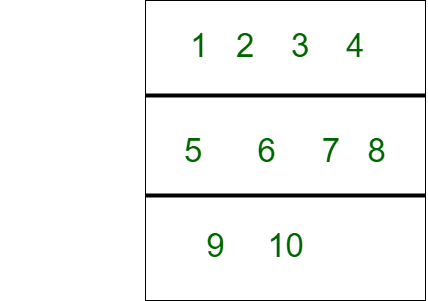# Cache Hits in Memory Organization

• Difficulty Level : Expert
• Last Updated : 01 May, 2022

The user has a memory machine. It has one layer for data storage and another layer for the cache. The user has stored an array with length N in the first layer. When the CPU needs data, it immediately checks in cache memory whether it has data or not. If data is present it results in CACHE HITS, else CACHE MISS, i.e., data is not in cache memory so it retrieves data from main memory and inserts a block of data into the cache layer.The question that arises is: How many times machine will need to load a block into the cache layer, i.e. determine the number of CACHE MISS?

Example

Let us assume an array and denote its elements by A0, A1, …, AN? Now user wants to load some elements of this array into the cache.Machine loads array in blocks with size B:
Assume block size is 4.
1 2 3 4 comes in Block 1, 5 6 7 8 comes in Block 2 and 9,10 comes in Block 3.A0, A1, …, AB? 1 form a block; AB, AB+1, … , A2B? 1 form another block, and so on. The last block may contain less than B elements of user’s array.
Cache may only contain at most one block at a time. Whenever user tries to access an element Ai, machine checks if block where Ai is located is already in cache, and if it is not, loads this block into cache layer, so that it can quickly access any data in it.
However, as soon as user tries to access an element that is outside currently loaded block in cache, block that was previously loaded into cache is removed from cache, since machine loads a new block containing element that is being accessed.

Example –
User has a sequence of elements Ax1, Ax2, …, AxM which he wants to access, in this order. Initially, cache is empty. We need to find out how many times machine will need to load a block into cache layer.

Input Format :

• First line of each test case contains three space-separated integers N, B and M.
• Second line contains M space-separated integers x1, x2, …, xM.

Input :

```5 3 3
0 3 4```

Output :

`2`

Explanation :
Machine stores elements [A0, A1, A2] in one block and [A3, A4] in another block. When accessing A0, block [A0, A1, A2] is loaded. Then, accessing A3 removes previous block from cache and loads block [A3, A4]. Finally, when user accesses A4, a new block is not loaded, since block containing A4 is currently loaded in cache.

Approach :

• Initially cache miss occurs because cache layer is empty and we find next multiplier and starting element.
• Obtain user value and find next multiplier number which is divisible by block size.
• Find starting elements of current block.
• If user value is greater than next multiplier and lesser than starting element then cache miss occurs.

Implementation :

## C++

 `// C++ program to implement Cache Hits``#include ``using` `namespace` `std;` `// Function to find the next multiplier``int` `findnextmultiplier(``int` `i, ``int` `b)``{``    ``for` `(``int` `j = i; j <= i * b; j++) {``        ``if` `(j % b == 0)``            ``return` `j;``    ``}``}` `// Function to find the cache hits``int` `ans(``int` `n, ``int` `b, ``int` `m, ``int` `user[])``{``   ``// Initially cache miss occurs``   ``int` `start, cacheMiss = 1, nextmultiplier;` `    ``// Find next multiplier for ith user and start``    ``nextmultiplier= findnextmultiplier(user + 1, b);``    ``start = nextmultiplier - b + 1;` `    ``for` `(``int` `i = 1; i < m; i++) {``        ``// If ith user is greater than nextmultiplier or lesser ``        ``// than start then cache miss occurs``        ``if` `(user[i] + 1 > nextmultiplier || user[i] + 1 < start) {``            ``cacheMiss++;``            ``nextmultiplier= findnextmultiplier(user[i] + 1, b);``            ``start = nextmultiplier - b + 1;``        ``}``    ``}` `    ``// Printing cache hits``    ``cout << cacheMiss << endl;`` ``return` `0;``}` `// Driver code``int` `main()``{``    ``int` `n=5, b=3, m=3;``    ``int` `user = {0, 3, 4};``    ``ans(n, b, m, user);``   ``return` `0;``}`

## Java

 `// Java program to implement Cache Hits``public` `class` `Main``{``    ``// Function to find the next multiplier``    ``public` `static` `int` `findnextmultiplier(``int` `i, ``int` `b)``    ``{``        ``for` `(``int` `j = i; j <= i * b; j++)``        ``{``            ``if` `(j % b == ``0``)``                ``return` `j;``        ``}``        ` `        ``return` `0``;``    ``}``      ` `    ``// Function to find the cache hits``    ``public` `static` `int` `ans(``int` `n, ``int` `b, ``int` `m, ``int` `user[])``    ``{``      ` `       ``// Initially cache miss occurs``       ``int` `start, ch = ``1``, nextmultiplier;``      ` `        ``// Find next multiplier for ith user and start``        ``nextmultiplier= findnextmultiplier(user[``0``] + ``1``, b);``        ``start = nextmultiplier - b + ``1``;``      ` `        ``for` `(``int` `i = ``1``; i < m; i++)``        ``{``          ` `            ``// If ith user is greater than nextmultiplier or lesser  ``            ``// than start then cache hit occurs``            ``if` `(user[i] + ``1` `> nextmultiplier || user[i] + ``1` `< start)``            ``{``                ``ch++;``                ``nextmultiplier= findnextmultiplier(user[i] + ``1``, b);``                ``start = nextmultiplier - b + ``1``;``            ``}``        ``}``      ` `        ``// Printing cache hits``        ``System.out.println(ch);``     ``return` `0``;``    ``}` `    ``public` `static` `void` `main(String[] args)``    ``{``        ``int` `n=``5``, b=``3``, m=``3``;``        ``int` `user[] = {``0``, ``3``, ``4``};``        ``ans(n, b, m, user);``    ``}``}` `// This code is contributed by divyeshrabadiya07`

## Python3

 `# Python3 program to implement Cache Hits` `# Function to find the next multiplier``def` `findnextmultiplier(i, b):``    ` `    ``for` `j ``in` `range``(i, (i ``*` `b) ``+` `1``):``        ``if` `(j ``%` `b ``=``=` `0``):``            ``return` `j` `# Function to find the cache hits``def` `ans(n, b, m, user):``    ` `    ``# Initially cache hit occurs``    ``ch ``=` `1` `    ``# Find next multiplier for ith user and start``    ``nextmultiplier ``=` `findnextmultiplier(user[``0``] ``+` `1``, b)``    ``start ``=` `nextmultiplier ``-` `b ``+` `1` `    ``for` `i ``in` `range``(``1``, m):``        ` `        ``# If ith user is greater than nextmultiplier``        ``# or lesser than start then cache hit occurs``        ``if` `(user[i] ``+` `1` `> nextmultiplier ``or``            ``user[i] ``+` `1` `< start):``            ``ch ``+``=` `1``            ``nextmultiplier ``=` `findnextmultiplier(``                ``user[i] ``+` `1``, b)``            ``start ``=` `nextmultiplier ``-` `b ``+` `1` `    ``# Printing cache hits``    ``print``(ch)` `# Driver code``n ``=` `5``b ``=` `3``m ``=` `3` `user ``=` `[ ``0``, ``3``, ``4` `]``ans(n, b, m, user)` `# This code is contributed by rag2127`

## C#

 `// C# program to implement Cache Hits``using` `System;` `class` `GFG{``    ` `// Function to find the next multiplier``static` `int` `findnextmultiplier(``int` `i, ``int` `b)``{``    ``for``(``int` `j = i; j <= i * b; j++)``    ``{``        ``if` `(j % b == 0)``            ``return` `j;``    ``}``    ``return` `0;``}``   ` `// Function to find the cache hits``static` `int` `ans(``int` `n, ``int` `b, ``int` `m, ``int``[] user)``{``    ` `    ``// Initially cache hit occurs``    ``int` `start, ch = 1, nextmultiplier;``    ` `    ``// Find next multiplier for ith user and start``    ``nextmultiplier = findnextmultiplier(``                   ``user + 1, b);``    ``start = nextmultiplier - b + 1;``    ` `    ``for``(``int` `i = 1; i < m; i++)``    ``{``        ` `        ``// If ith user is greater than nextmultiplier``        ``// or lesser than start then cache hit occurs``        ``if` `(user[i] + 1 > nextmultiplier ||``            ``user[i] + 1 < start)``        ``{``            ``ch++;``            ``nextmultiplier = findnextmultiplier(``                           ``user[i] + 1, b);``            ``start = nextmultiplier - b + 1;``        ``}``    ``}``    ` `    ``// Printing cache hits``    ``Console.WriteLine(ch);``    ``return` `0;``}` `// Driver Code ``static` `void` `Main()``{``    ``int` `n = 5, b = 3, m = 3;``    ``int``[] user = { 0, 3, 4 };``    ` `    ``ans(n, b, m, user);``}``}` `// This code is contributed by divyesh072019`

## Javascript

 ``

Output :

`2`

Time Complexity : O(m)
Auxiliary Space : O(1)

My Personal Notes arrow_drop_up